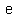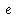# U+212F SCRIPT SMALL E

U+212F was added to Unicode in version 1.1 (1993). It belongs to the blockLetterlike Symbols in the Basic Multilingual Plane.

This character is a Lowercase Letter and is commonly used, that is, in no specific script. The character is also known as error and natural exponent.

The glyph is a Font composition of the glyphs. It has a Neutral East Asian Width. In bidirectional context it acts as Left To Right and is not mirrored. The glyph can, under circumstances, be confused with 29 other glyphs. In text U+212F behaves as Alphabetic regarding line breaks. It has type Lower for sentence and ALetter for word breaks. The Grapheme Cluster Break is Any.

The number e is an important mathematical constant that is the base of the natural logarithm. It is approximately equal to 2.71828, and is the limit of (1 + 1/n)n as n approaches infinity, an expression that arises in the study of compound interest. It can also be calculated as the sum of the infinite series

The constant can be defined in many ways. For example, e can be defined as the unique positive number a such that the graph of the function y = ax has unit slope at x = 0. The function f(x) = ex is called the exponential function, and its inverse is the natural logarithm, or logarithm to base e. The natural logarithm of a positive number k can also be defined directly as the area under the curve y = 1/x between x = 1 and x = k, in which case, e is the number whose natural logarithm is 1. There are alternative characterizations.

Sometimes called Euler's number after the Swiss mathematician Leonhard Euler, e is not to be confused with γ—the Euler–Mascheroni constant, sometimes called simply Euler's constant. The number e is also known as Napier's constant, but Euler's choice of the symbol e is said to have been retained in his honor. The constant was discovered by the Swiss mathematician Jacob Bernoulli while studying compound interest.

The number e is of eminent importance in mathematics, alongside 0, 1, π and i. All five of these numbers play important and recurring roles across mathematics, and are the five constants appearing in one formulation of Euler's identity. Like the constant π, e is irrational: it is not a ratio of integers; and it is transcendental: it is not a root of any non-zero polynomial with rational coefficients. The numerical value of e truncated to 50 decimal places is

2.71828182845904523536028747135266249775724709369995... (sequence A001113 in OEIS).

## Representations

System Representation
8495
UTF-8 E2 84 AF
UTF-16 21 2F
UTF-32 00 00 21 2F
URL-Quoted %E2%84%AF
HTML-Escape &#x212F;
Wrong windows-1252 Mojibake â¯
HTML-Escape &escr;
alias error
alias natural exponent
LaTeX \mathscr{e}

## Complete Record

Property Value
Age (age) 1.1
Unicode Name (na) SCRIPT SMALL E
Unicode 1 Name (na1)
Block (blk) Letterlike_Symbols
General Category (gc) Lowercase Letter
Script (sc) Common
Bidirectional Category (bc) Left To Right
Combining Class (ccc) Not Reordered
Decomposition Type (dt) Font
Decomposition Mapping (dm)Lowercase (Lower)
Simple Lowercase Mapping (slc)Lowercase Mapping (lc)Uppercase (Upper)
Simple Uppercase Mapping (suc)Uppercase Mapping (uc)Simple Titlecase Mapping (stc)Titlecase Mapping (tc)Case Folding (cf)ASCII Hex Digit (AHex)
Alphabetic (Alpha)
Bidi Control (Bidi_C)
Bidi Mirrored (Bidi_M)
Bidi Paired Bracket (bpb)Bidi Paired Bracket Type (bpt) None
Cased (Cased)
Composition Exclusion (CE)
Case Ignorable (CI)
Full Composition Exclusion (Comp_Ex)
Changes When Casefolded (CWCF)
Changes When Casemapped (CWCM)
Changes When NFKC Casefolded (CWKCF)
Changes When Lowercased (CWL)
Changes When Titlecased (CWT)
Changes When Uppercased (CWU)
Dash (Dash)
Deprecated (Dep)
Default Ignorable Code Point (DI)
Diacritic (Dia)
East Asian Width (ea) Neutral
Extender (Ext)
FC NFKC Closure (FC_NFKC)Grapheme Cluster Break (GCB) Any
Grapheme Base (Gr_Base)
Grapheme Extend (Gr_Ext)
Hex Digit (Hex)
Hangul Syllable Type (hst) Not Applicable
Hyphen (Hyphen)
ID Continue (IDC)
Ideographic (Ideo)
ID Start (IDS)
IDS Binary Operator (IDSB)
IDS Trinary Operator and (IDST)
InMC (InMC)
Indic Positional Category (InPC) NA
Indic Syllabic Category (InSC) Other
ISO 10646 Comment (isc)
Joining Group (jg) No_Joining_Group
Join Control (Join_C)
Jamo Short Name (JSN)
Joining Type (jt) Non Joining
Line Break (lb) Alphabetic
Logical Order Exception (LOE)
Math (Math)
Noncharacter Code Point (NChar)
NFC Quick Check (NFC_QC) Yes
NFD Quick Check (NFD_QC) Yes
NFKC Casefold (NFKC_CF)NFKC Quick Check (NFKC_QC) No
NFKD Quick Check (NFKD_QC) No
Numeric Type (nt) None
Numeric Value (nv) NaN
Other Alphabetic (OAlpha)
Other Default Ignorable Code Point (ODI)
Other Grapheme Extend (OGr_Ext)
Other ID Continue (OIDC)
Other ID Start (OIDS)
Other Lowercase (OLower)
Other Math (OMath)
Other Uppercase (OUpper)
Pattern Syntax (Pat_Syn)
Pattern White Space (Pat_WS)
Quotation Mark (QMark)
Sentence Break (SB) Lower
Simple Case Folding (scf)Script Extension (scx) Common
Soft Dotted (SD)
STerm (STerm)
Terminal Punctuation (Term)
Unified Ideograph (UIdeo)
Variation Selector (VS)
Word Break (WB) ALetter
White Space (WSpace)
XID Continue (XIDC)
XID Start (XIDS)
Expands On NFC (XO_NFC)
Expands On NFD (XO_NFD)
Expands On NFKC (XO_NFKC)
Expands On NFKD (XO_NFKD)